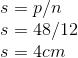## Example Questions

### Example Question #1 : How To Find The Length Of A Side Of A Polygon

Polygon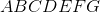is a regular seven-sided polygon, or heptagon, with perimeter 500. Which choice comes closest to the length of diagonal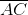?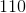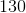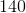Explanation:

Congruent sides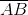and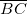and the diagonalform an isosceles triangle.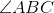, being an angle of a seven-sided regular polygon, has measure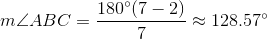The other two are congruent, and each has measure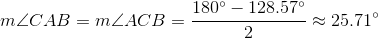The length of one side is one-seventh of 500, or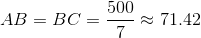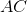can be found using the Law of Sines: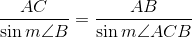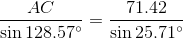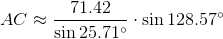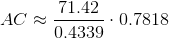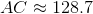Of the given choices, 130 comes closest.

### Example Question #2 : How To Find The Length Of A Side Of A Polygon

Polygon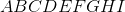is a regular nine-sided polygon, or nonagon, with perimeter 500. Which choice comes closest to the length of diagonal?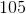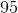Explanation:

Congruent sidesandand the diagonalform an isosceles triangle., being an angle of a nine-sided regular polygon, has measure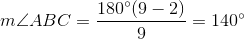The other two are congruent, and each has measure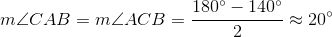The length of one side is one-ninth of 500, or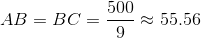can be found using the Law of Sines: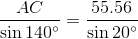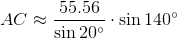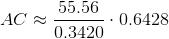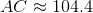Of the given choices, 105 comes closest.

### Example Question #3 : How To Find The Length Of A Side Of A Polygon

What is the side length of a 12-sided polygon with a perimeter of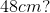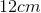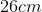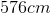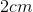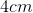To find the side length,, of an-sided polygon with a perimeter of.